# Excel String Functions – LEFT, RIGHT, MID, LEN, and FIND

• Last Updated : 21 Aug, 2021

Excel is mostly about numerical, however, we often have data of text data type. Here are a few functions we should know to handle text data.

• LEFT
• RIGHT
• MID
• LEN
• FIND

## The LEFT function

The LEFT function returns a given text from the left of our text string based on the number of characters specified.

Syntax:

`LEFT(text, [num_chars])`

Parameters:

• Text: The text we want to extract from.
• Num_chars (Optional): The number of characters you want to extract. Default num_chars is 1 and the number must be a positive number that is greater than zero.

Example:

Step 1: Format your data.

Now if you want to get the first “Geeks” from “Geeksforgeeks” in B2. Let us follow the next step.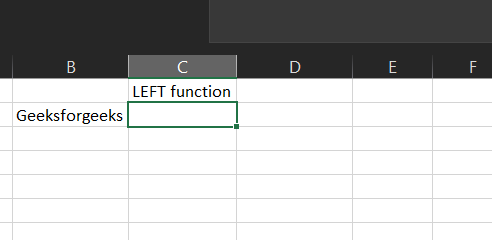Step 2: We will enter =LEFT(B2,5) in the B3 cell. Here we want Excel to extract the first 5 characters from the left side of our text in B2.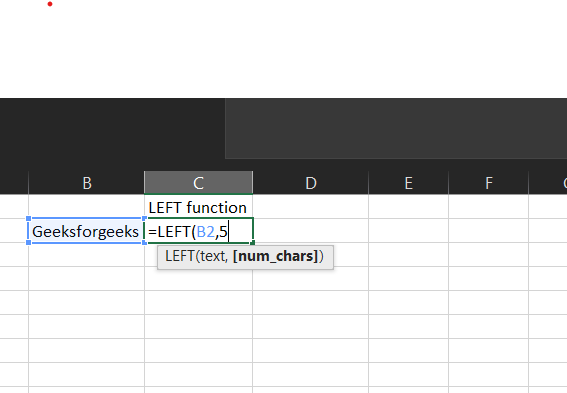This will return “Geeks”.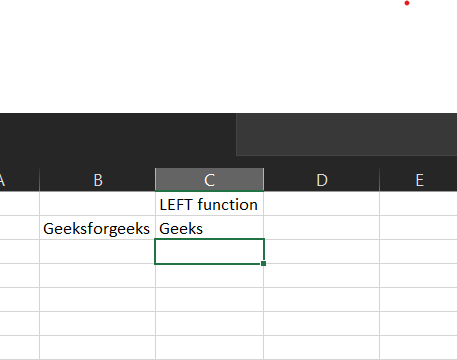## The RIGHT Function

The RIGHT function returns a given text from the left of our text string based on the number of characters specified.

Syntax:

`RIGHT(text, [num_chars])`

Parameters:

• Text: The text we want to extract from.
• Num_chars (Optional): The number of characters you want to extract. Default num_chars is 1 and the number must be a positive number that is greater than zero.

Example:

Step 1: Format your data.

Now if you want to get the last geeks from “Geeksforgeeks” in B2. Let us follow the next step.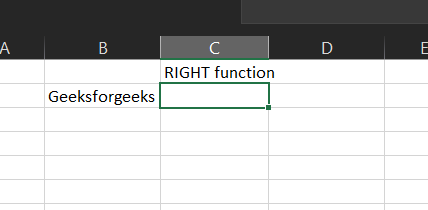Step 2: We will enter =RIGHT(B2,5) in the B3 cell. Here we want Excel to extract the last 5 characters from the right side of our text in B2.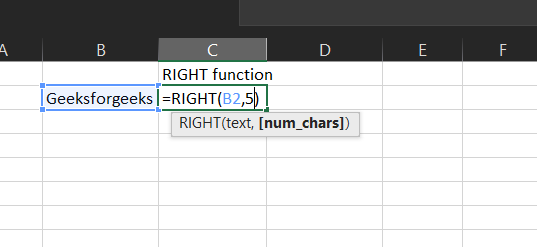This will return “geeks”.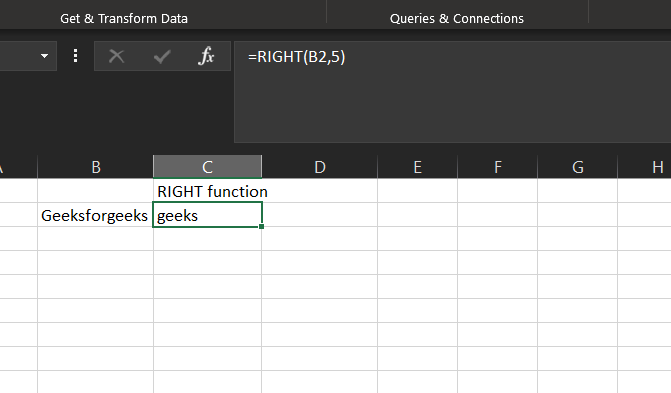## The MID function

The MID function returns the text from any middle part of our text string based on the starting position and number of characters specified.

Syntax:

`MID(text, start_num, num_chars)`

Parameters:

• Text: The text we want to extract from.
• start_num: The starting number of the first character from the text we want to extract.
• Num_chars: The number of characters you want to extract.

Example:

Step 1: Format your data.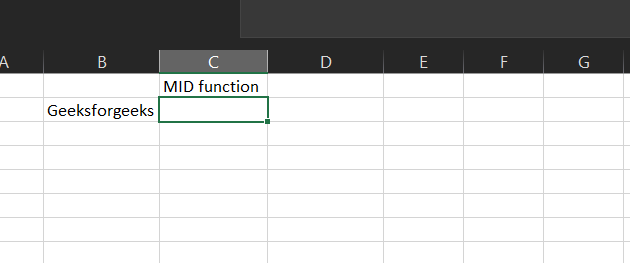Now if you want to get the character “for” which is located in the middle of our text “Geeksforgeeks” in B2. Let us follow the next step.

Step 2: We will enter =MID(B2,5,3) in the B3 cell. Here we want Excel to extract the characters located in the middle of our text in B2.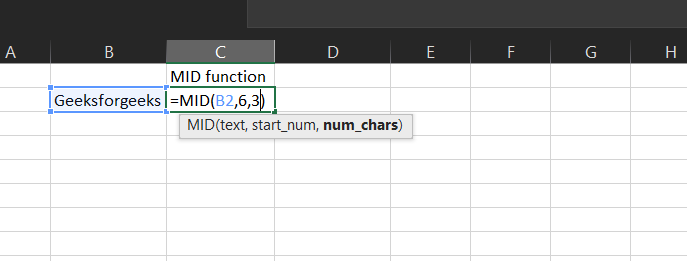This will return “for”.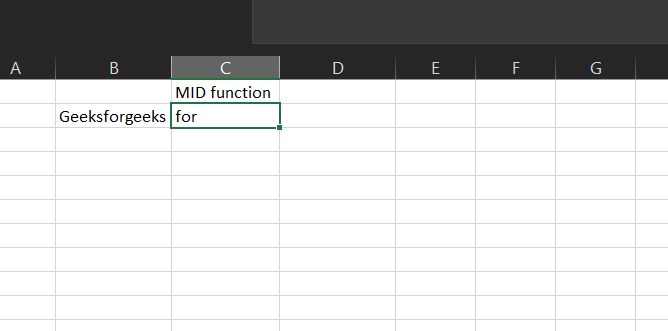## The Len Function

The LEN function returns the number of characters in the text strings.

Syntax:

`LEN(text)`

Parameters:

• Text: The text we want to know the length

Example:

Step 1: Format your data.

Now if you want to know how many characters are in the text “Geeksforgeeks”. Let us follow the next step.Step 2: We will enter =LEN(B2) in the B3 cell. Excel will count how many characters are in the text.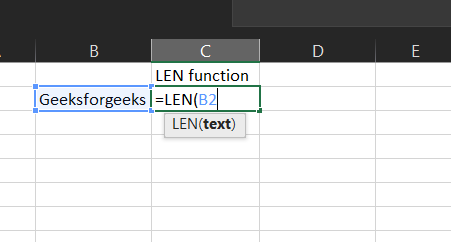This will return 13.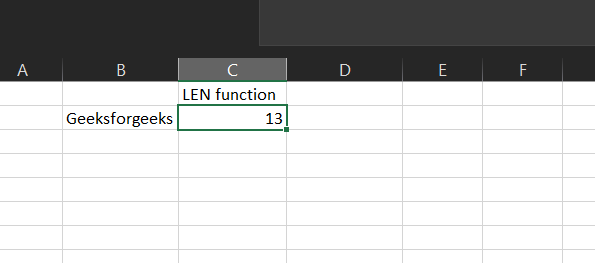## The FIND Function

The FIND function returns the position of a given text within a text.

Syntax:

`FIND(find_text, within_text, [start_num])`

Parameters:

• Find_text: The text we want to find.
• Within_text: The text containing our find_text.
• Start_num (Optional): The starting position of our find_text. Default is 1.

Example:

Step 1: Format your data.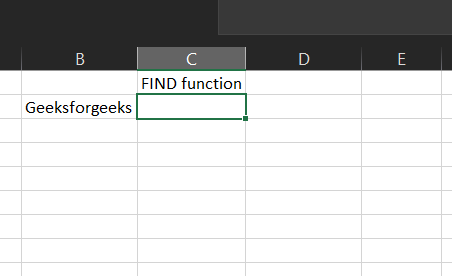Now if you want to find “for” in geeksforgeeks in B2. Let us follow the next step.

Step 2: We will enter =FIND(“for”,B2) in B3 cell. Here we want Excel to find “for” in our text in B2.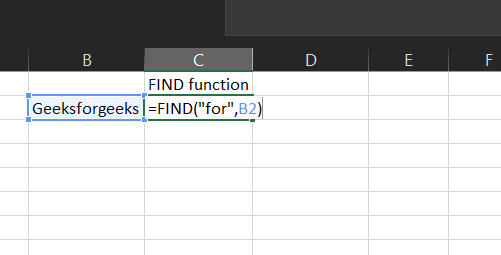This will return 6 because “for” is located at character number 6 in our text.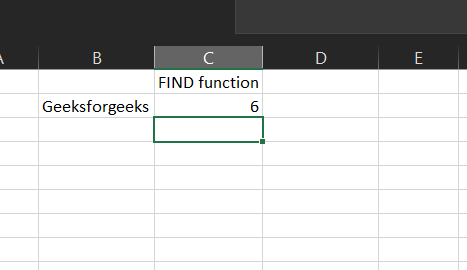My Personal Notes arrow_drop_up
Recommended Articles
Page :